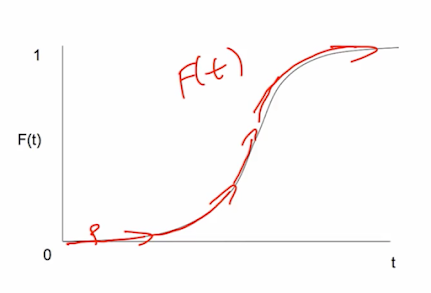One thing about the Bass Model is networks are not going to make on explicit appearance, we'll enrich the analysis by bringing interaction structure in explicitly, and try to understand how things diffuse over time.

# Bass Model¶

• A benchmark model with no explicit social structure

• Two actions/states/behaviors 0 and 1

• F(t) fraction of the population who have adopted action 1 at time t

• p: rate of spontaneous innovation/adoption

• that's going to be a rate that's independent of what else is going on in the economy or the world or the society. So some people will just go and decide to see a movie regardless of what's going on, or they will adopt corn, regardless of whether other people are doing it, or they've heard about it.
• q: rate of imitation of adoption

• And there's some other individuals who will actually do it as a function of having heard about it, or somehow imitated other individuals who were doint it.
• $dF(t)/dt = (p + q F(t))(1 - F(t))$

• $(1 - F(t))$ are the people who have not yet adopted yet moved to "1", so they haven't become a "1" yet so they're still "0". So $(1 - F(t))$ are the people who are still "0" and can possibly change. And then the fraction of those people change directly spontaneously, that's the "p". And the others change by imitating the existing population. So you have a chance of just spontaneously deciding to become a "1", or you also have a chance of imitating somebody in the population and that's proportional to how many in the population are already "1", so this "q" parameter says that you have some rate of imitation
• $F(t) = (1 - e^{-(p+q)t}) / (1 + pe^{-(p+q)t}/q)$

• So higher p and q are going to lead to faster diffusion, so more people adopting by any particular time, lower

# Getting the S-shape¶

• Gives S-shape (if q > p) and tends to "1" in the limit as t becomes large

• Initially only "p" matters, then "q" takes over

• Eventually change slows as F(t) approaches 1

$$dF(t)/dt = (p + q F(t))(1 - F(t))$$

• when F(t) nears 1, $dF(t)/dt = 0$

• when F(t) = 0, $dF(t)/dt = p$

• when F(t) = $\epsilon$, $dF(t)/dt = (p + q\epsilon)(1 - \epsilon)$

• to get initial convexity: need $(p + q\epsilon)(1 - \epsilon) > p$

• $q(1 - \epsilon) > p$, so initially need $q > p$# Next: Beyond Bass - Component Structure¶

• Reach of diffusion is bounded by the component structure

• Some players or nodes are immune

• Some links fail to transmit...

• Answers questions of when get diffusion, and its extent (neither answered by simple Bass)